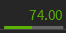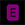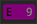Wondering what all the different columns in the Positions tab mean? Below is a glossary of each column that explains what each one displays.

Looking to add any of the columns listed below to your Positions tab? To learn how to add or remove columns in your Positions tab, click here.

Beta

Measures the movement of a stock’s price in relation to the overall market (in most cases, the S&P 500, or SPX, serves as a stand-in for the overall market). Example: XYZ is trading at \$100 and has a beta of 0.80. If the market moves up by 1 then, theoretically, XYZ will move up by \$0.80. Conversely, if the market moves down then, theoretically, XYZ will move down by -\$0.80.

Beta Weighted Net Delta

The theoretical movement of the position given a \$1 move up in the SPY (the default Beta Weight of the platform). This metric can help you measure the amount of risk in your position. It essentially provides an “apples-to-apples”. To learn more about beta weighting then click here.

Capital Requirement (Cap Req)

Close Price

The closing price of stock or option on the last trading day. It is the base price for the “P/L Day” metric.

Cost

The cost of the trade (gross, without commissions and fees). This number is fixed. It is best to interpret the Positions tab as displaying money inflows and outflows. If you are short a position, then you will see a green (positive) number from the proceeds you received for shorting a stock or options position. On the flip side, if you are long a position then you will see a red (negative) number because you had to pay that amount to be in that position.

Days to Expiration (DTE)

The column will display the number of days until expiration. This column may be sorted in ascending or descending order by clicking the column header to help with identifying positions that are near expiration.

Days Since Open (D's Open)

The number of days a position is open in your portfolio.

Delta Per Qty

The delta amount before the options multiplier (100). For example, if an option has a 30 delta then the Delta Per Qty will be 0.3 (30 Delta / 100 multiplier = 0.3 Delta Per Qty). It is reflected the same way as if you were viewing the option within the Table view of the Trade Tab (options chain). To learn more about delta, then click here.

Equity Probability of Profit (ePoP)

The Equity Probability of Profit (ePoP) is theoretical probability of profit of your portfolio's equity/etf positions (stock & options) making at least \$0.01. ePoP does not apply to futures or futures options positions. To learn more about ePoP, please click here.

Ext

The amount of extrinsic value of an option based from the mid-price. If an option is out-of-the-money, then the mid-price of the option will be the EXT value since out-of-the-money options are based solely on extrinsic value. On the other hand, if an option was in-the-money then the EXT will show the extrinsic value an option has. For example, if an option is \$1 in-the-money, but the option is marking at \$1.20 (mid-price) then the EXT for that in-the-money option will be \$20. EXT indicates how much extrinsic value, or time value, an option position has. Long options will display as a negative number and short options will display as a positive number.

Gamma Per Qty

The gamma amount before the options multiplier (100). For example, if an option has a gamma of 11 then the Gamma Per Qty will be 0.11 (11 Gamma / 100 multiplier = 0.11 Gamma Per Qty). It is reflected the same way as if you were viewing the option within the Table view of the Trade Tab (options chain). To learn more about gamma, then click here.

Mark

The value that the platform displays as the price of stock or option. It is typically the mid-price of stock or option. It is also the metric the platform uses for profit/loss calculations. To see how mid-price calculation, then please view “Quote Mid.”

Net Delta

The total deltas of an option after the multiplier (100). For example, if you sell a put that had a .30 delta then that means it will have a net delta of 30 (.30 Delta Per Qty x 100 = 30 Net Deltas). To learn more about delta, then click here.

Net Gamma

The total gamma of an option after the multiplier (100). For example, if you sell a put that had a .11 gamma then that means it will have a net gamma of 11 (.11 Gamma Per Qty x 100 = 11 Net Gamma). To learn more about gamma, then click here.

Net Liq

Net Liq is short for “Net Liquidation Value.” Net Liq is calculated from the mid-price of the position. This number is dynamic and will move according to the market price.

Net Theta

The total theta of an option after the multiplier (100). For example, if you sell a put that had a .15 theta then that means it will have a positive net theta of 15 (.15 Theta Per Qty x 100 Net Gamma = 15 Net Theta). To learn more about theta, then click here.

Net Vega

The total vega of an option after the multiplier (100). Vega values represent the change in an option’s price given a 1% move in implied volatility, all else equal. For example, if you sell a put that had a -0.05 Vega then that means it will have a negative net vega of -5 (-.05 Vega Per Qty x 100 Net Gamma = -5 Net Vega). To learn more about vega then click here.

P/L Change

The overall percentage return/loss on a position. This will show what your profit or loss is in a percentage. For example, if you sold a put and collected \$1.00 credit (\$100 after options multiplier) and it was current marking at a price \$0.50 (\$50 after options multiplier) then that put would be marked at 50% return.

P/L Day

The nominal profit or loss in a day based on the prior day’s close. The calculation is based on the closing price of the stock or option and will change according to the price action of the stock or option. Each day it will start from \$0.00 and will move positive or negative. For example, a long option increases in price by \$0.25 will display a P/L Day of \$25.

P/L Day Change

The percentage profit or loss in a day based on the prior day’s close. The calculation is based on the closing price of the stock or option and will change according to the price action of the stock or option. Each day it will start from 0.0% and will move positive or negative. For example, a long option that closed at \$1.00 on the last trading day increases \$0.25 today. The current mark for the option is \$1.25 and will display a P/L Day Change of 25%.

P/L Day Gain Per Qty

The total profit or loss in a given day on a per quantity basis. This means the metric will show an options profit before the multiplier of 100. For stock, it will simply be based on the price movement on a per share basis, whether you are long or short. The calculation is based on the closing price of the stock or option from the last trading day.

P/L Day w/ Percent Bar

Please refer to the entry for “Percent Bar”.

P/L Open

The total nominal profit or loss from the time that a position was opened.

P/L Open Per Qty

The total gain or loss on a given day, on a per quantity basis, with the calculation based on the opening price of the stock or option.

P/L w/ Percent Bar

The total nominal profit or loss from the time that an option or stock position was opened. To read about the colored bar beneath P/L Open, refer to the entry for “Percent Bar”.

Percent Bar (located beneath P/L w/ Percent Bar and P/L day w/ Percent Bar)

The bar beneath your P/L Day or P/L Open number is your percentage bar. It is a quick visual reference for the percentage profit or loss from a position. Here is an example of a position marking at a 46.8% profit. You will notice that the green bar below is filled nearly halfway. When a percent bar is fully filled a green or red dot will appear to the right of the bar. The dot denotes a profit (green) or loss (red) greater than 100%.

Let's break down an example:

-XYZ is trading at \$100

-Day 1: Let's say we sold an XYZ \$95 naked put for \$1.00

-Day 2: The next day XYZ rallies up to \$120, and the put is trading at \$0.50. The bar below your P/L would be filled-in green to the halfway point, which represents a 50% P/L Open. At that point, you can either close out the position or keep it on - let’s keep it on for the sake of this example.

-Day 3: XYZ moves down to \$85. The stock has blown through your break-even of \$99, and you're now facing a full loss of \$1.00 + intrinsic of \$15 + Time Premium. Let's just say that the put is now trading at \$16. Since your put is now at a full loss, plus some, the percentage bar below will be fully filled-in red.

-Day 4: XYZ recovers and returns to \$100; the put is now trading at \$1. The percentage bar below P/L should now just be an empty grey bar. The P/L is now \$0 (or 0%), so the entire bar is just empty/grey.

You may also add a “P/L Change” column within the Positions tab. It will show the actual % of P/L. You can arrange it so that it's next to your “P/L Open” and you should see that the P/L Change metric corresponds to the percentage bar.

PoP

POP stands for the probability of profit. This is the probability that you have of making at least \$0.01 on the trade at expiration. For trades performed at a credit, it's the likelihood of the break-even price expiring out-of-the-money, and for trades executed at a debit, it's the probability of the break-even price expiring in-the-money.

The lowest current asking price for the position.

Quote Bid

The highest current bid price for the position.

Quote Last

The last price the position was traded at.

Quote Mid

As the name implies, it is the mid-point price between the bid and ask. It is a theoretical price and not a guaranteed price. It can aid in price discovery. The formula for the mid-price is: Mid Price = ((Ask-Bid) / 2) + Bid.

Realized Day Gain

An aggregate amount of profit or loss on a realized (already closed) position for the current trading day. This number is based on the position's opening mark for the day.

Realized Today

The total profit or loss realized from a position since it was opened.

Theta Per Qty

The theta amount before the options multiplier (100). For example, if an option has a theta of 2 then the Theta Per Qty will be 0.02 (2 theta / 100 multiplier = 0.02 Theta Per Qty). It is reflected the same way as if you were viewing the option within the Table view of the Trade Tab (options chain). To learn more about theta then click here.

The price at which a position was opened at. If enabled, the trade price of an underlying with options positions will be added when grouped. The individual trade price of each option will be displayed when the symbol group expanded.

Underlying Indicators

Notable upcoming events relating to an underlying, such as an upcoming earnings date or dividend. Platform denotes upcoming earnings with a purple ‘E’ icon. If there is a published earnings date, then there will be an earnings countdown that will be next to the ‘E’. Dividends are denoted with a blue ‘D’ icon. Ex-dividend dates can be found under the ‘SYMBOL DETAIL’ located in the right sidebar menu.

Unrealized Day Gain

An aggregate amount of profit or loss on an unrealized (not closed) position for the current trading day.

Vega Per Qty

The vega amount before the options multiplier (100). Vega values represent the change in an option’s price given a 1% move in implied volatility, all else equal. For example, if an option has a vega of 3 then the Vega Per Qty will be 0.03 (3 vega / 100 multiplier = 0.03 Vega Per Qty). It is reflected the same way as if you were viewing the option within the Table view of the Trade Tab (options chain). All short options and spreads will have a negative vega. Meanwhile, all long options and spreads have positive vega. To learn more about Vega then click here.